JEE  >  Area of Bounded Region

# Area of Bounded Region | Mathematics (Maths) Class 12 - JEE

 1 Crore+ students have signed up on EduRev. Have you?

The space occupied by the curve along with the axis, under the given condition is called area of bounded region.

(i) The area bounded by the curve y = F(x) above the X-axis and between the lines x = a, x = b is given by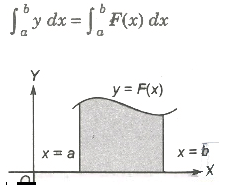(ii) If the curve between the lines x = a, x = b lies below the X-axis, then the required area is given by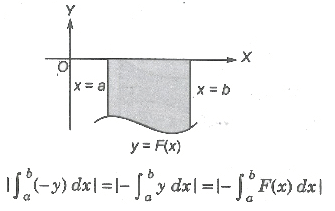(iii) The area bounded by the curve x = F(y) right to the Y-axis and the lines y = c, y = d is given by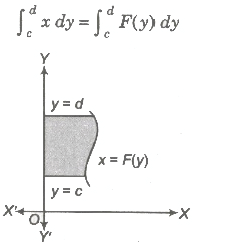(iv) If the curve between the lines y = c, y = d left to the Y-axis, then the area is given by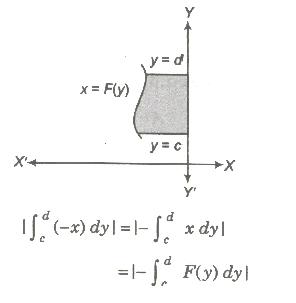(v) Area bounded by two curves y = F (x) and y = G (x) between x = a and x = b is given by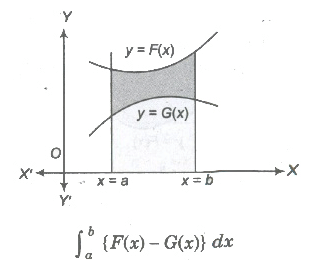(vi) Area bounded by two curves x = f(y) and x = g(y) between y=c and y=d is given by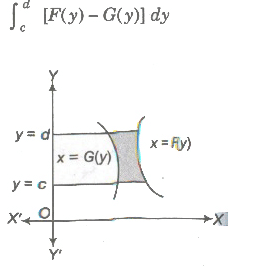(vii) If F (x) ≥. G (x) in [a, c] and F (x) ≤ G (x) in [c,d], where a < c < b, then area of the region bounded by the curves is given as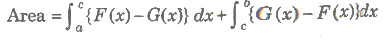Area of Curves Given by Polar Equations

Let  f(θ) be a continuous function,  θ ∈ (a, α), then the are t bounded by the curve r = f(θ) and radius α, β(α < β) is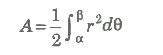Area of Parametric Curves

Let x = φ(t) and y = ψ(t) be two parametric curves, then area bounded by the curve, X-axis and ordinates x = φ(t1), x = ψ(t2) is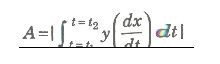Volume and Surface Area

If We revolve any plane curve along any line, then solid so generated is called solid of revolution.

1. Volume of Solid Revolution

1. The volume of the solid generated by revolution of the area bounded by the curve y = f(x), the axis of x and the ordinates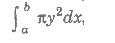it being given that f(x) is a continuous a function in the interval (a, b).

2. The volume of the solid generated by revolution of the area bounded by the curve x = g(y), the axis of y and two abscissas y = c and y = d is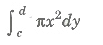it being given that g(y) is a continuous function in the interval (c, d).

Surface of Solid Revolution

(i) The surface of the solid generated by revolution of the area bounded by the curve y = f(x), the axis of x and the ordinates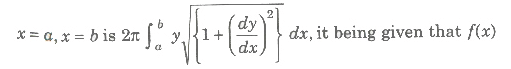is a continuous function in the interval (a, b).

(ii) The surface of the solid generated by revolution of the area bounded by the curve x = f (y), the axis of y and y = c, y = d is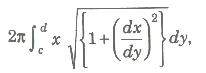continuous function in the interval (c, d).

Curve Sketching

1. symmetry

1. If powers of y in a equation of curve are all even, then curve is symmetrical about X-axis.
2. If powers of x in a equation of curve are all even, then curve is symmetrical about Y-axis.
3. When x is replaced by -x and y is replaced by -y, then curve is symmetrical in opposite quadrant.
4. If x and y are interchanged and equation of curve remains unchanged curve is symmetrical about line y = x.

2. Nature of Origin

1. If point (0, 0) satisfies the equation, then curve passes through origin.
2. If curve passes through origin, then equate low st degree term to zero and get equation of tangent. If there are two tangents, then origin is a double point.

3. Point of Intersection with Axes

1. Put y = 0 and get intersection with X-axis, put x = 0 and get intersection with Y-axis.
2. Now, find equation of tangent at this point i. e. , shift origin to the point of intersection and equate the lowest degree term to zero.
3. Find regions where curve does not exists. i. e., curve will not exit for those values of variable when makes the other imaginary or not defined.

4. Asymptotes

1. Equate coefficient of highest power of x and get asymptote parallel to X-axis.
2. Similarly equate coefficient of highest power of y and get asymptote parallel to Y-axis.

5. The Sign of (dy/dx)

Find points at which (dy/dx) vanishes or becomes infinite. It gives us the points where tangent is parallel or perpendicular to the X-axis.

6. Points of Inflexion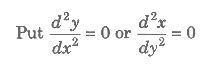and solve the resulting equation.If some point of inflexion is there, then locate it exactly.

Taking in consideration of all above information, we draw an approximate shape of the curve.

Shape of Some Curves is Given Below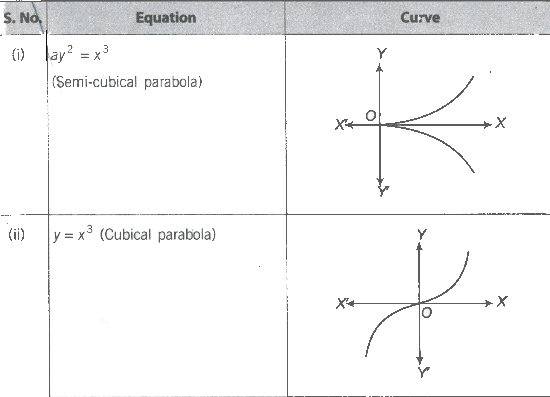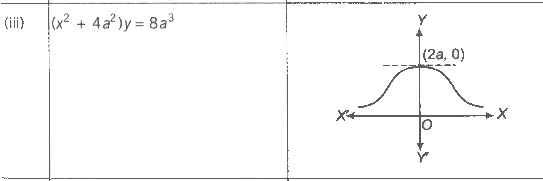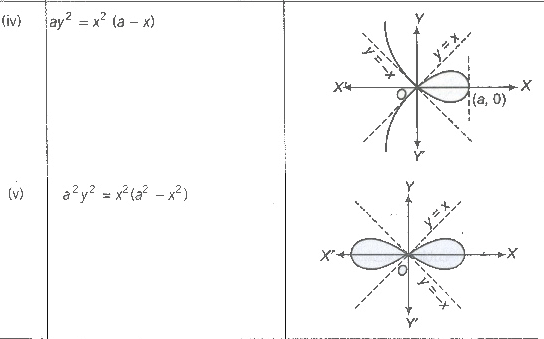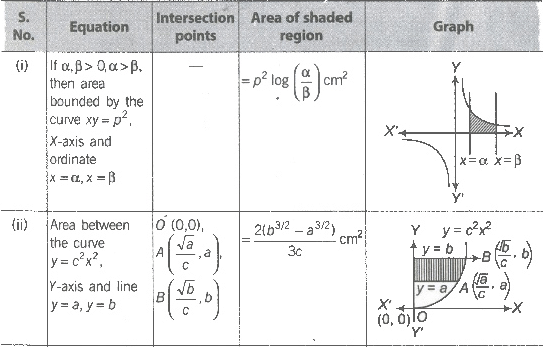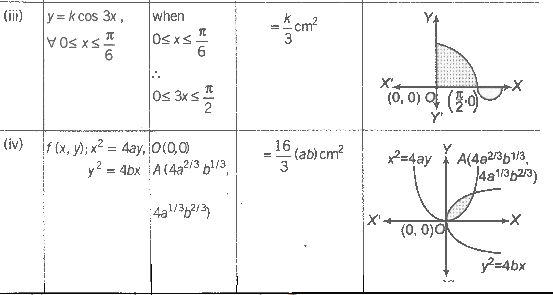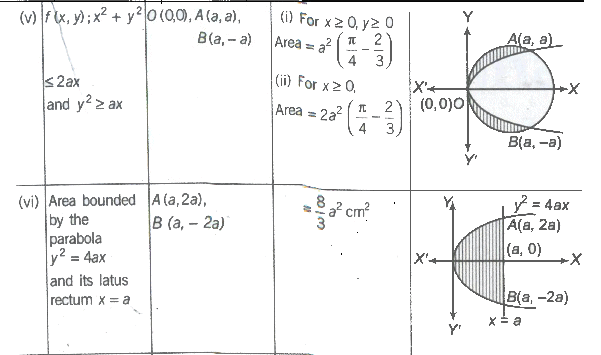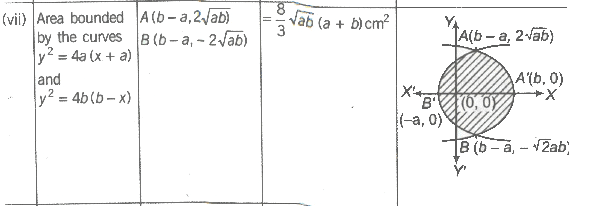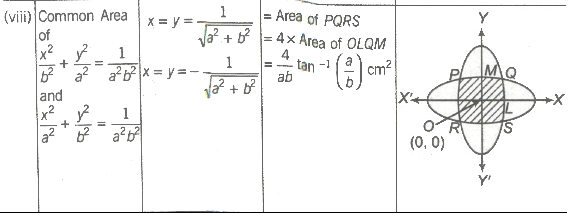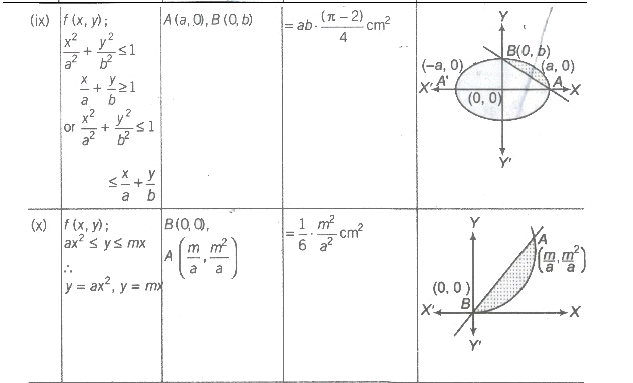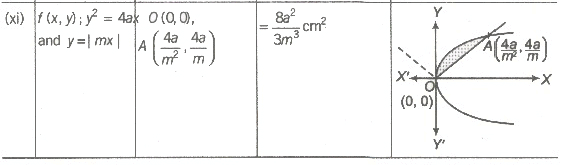The document Area of Bounded Region | Mathematics (Maths) Class 12 - JEE is a part of the JEE Course Mathematics (Maths) Class 12.
All you need of JEE at this link: JEE

## Mathematics (Maths) Class 12

209 videos|218 docs|139 tests

## Mathematics (Maths) Class 12

209 videos|218 docs|139 tests

Track your progress, build streaks, highlight & save important lessons and more!(Scan QR code)

,

,

,

,

,

,

,

,

,

,

,

,

,

,

,

,

,

,

,

,

,

;